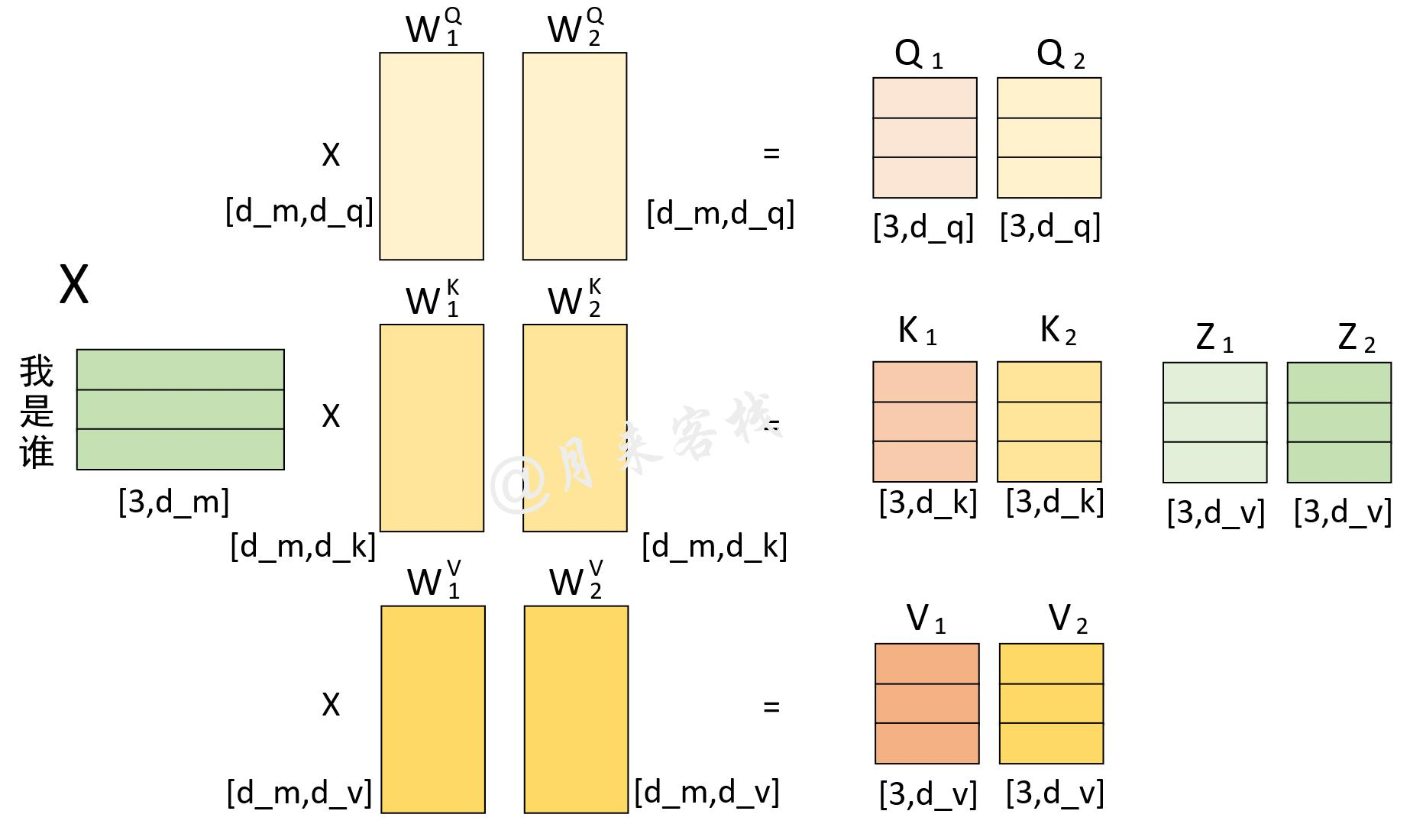# 1 引言

• 1 Transformer多头注意力机制的思想与原理；
• 2 Transformer的位置编码与编码解码过程；
• 3 Transformer的网络结构与自注意力机制实现；
• 4 Transformer的实现过程；
• 5 基于Transformer的翻译模型；
• 6 基于Transformer的文本分类模型；
• 7 基于Transformer的对联生成模型

# 2 动机

## 2.1 面临问题

This inherently sequential nature precludes parallelization within training examples, which becomes critical at longer sequence lengths, as memory constraints limit batching across examples.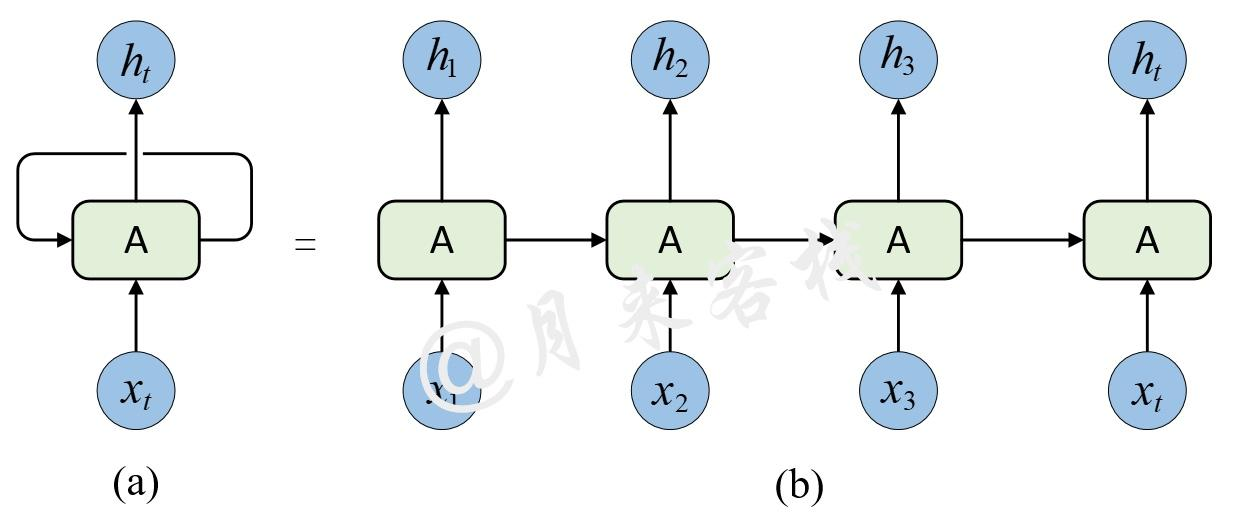Recent work has achieved significant improvements in computational efficiency through factorization tricks and conditional computation, while also improving model performance in case of the latter. The fundamental constraint of sequential computation, however, remains.

## 2.2 解决思路

To the best of our knowledge, however, the Transformer is the first transduction model relying entirely on self-attention to compute representations of its input and output without using sequence- aligned RNNs or convolution.

# 3 技术手段

## 3.1 self-Attention

An attention function can be described as mapping a query and a set of key-value pairs to an output, where the query, keys, values, and output are all vectors. The output is computed as a weighted sum of the values, where the weight assigned to each value is computed by a compatibility function of the query with the corresponding key.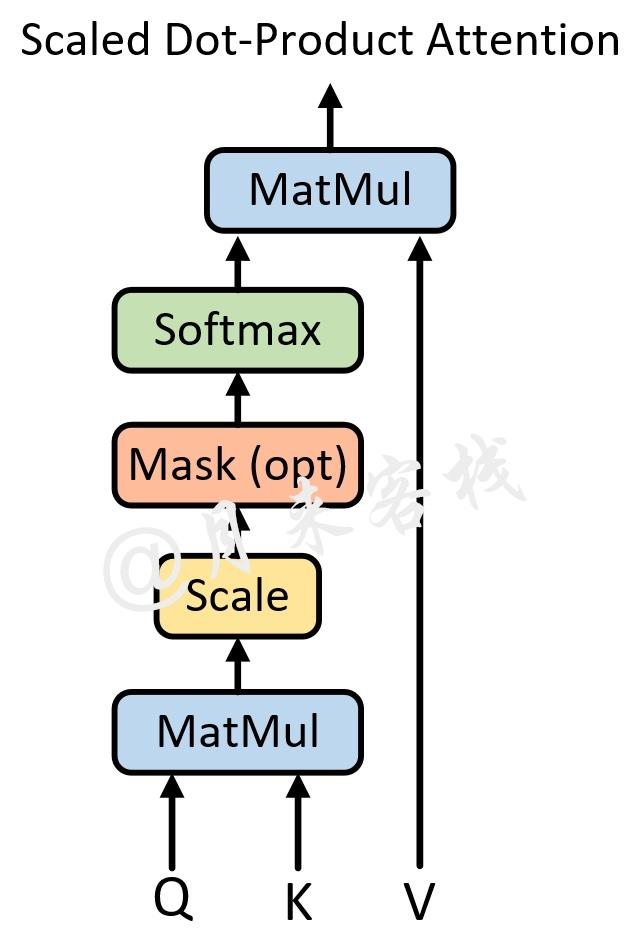Attention(Q,K,V)=softmax(\frac{QK^{T} }{\sqrt{d_{k}} } )V

We suspect that for large values of dk, the dot products grow large in magnitude, pushing the softmax function into regions where it has extremely small gradients.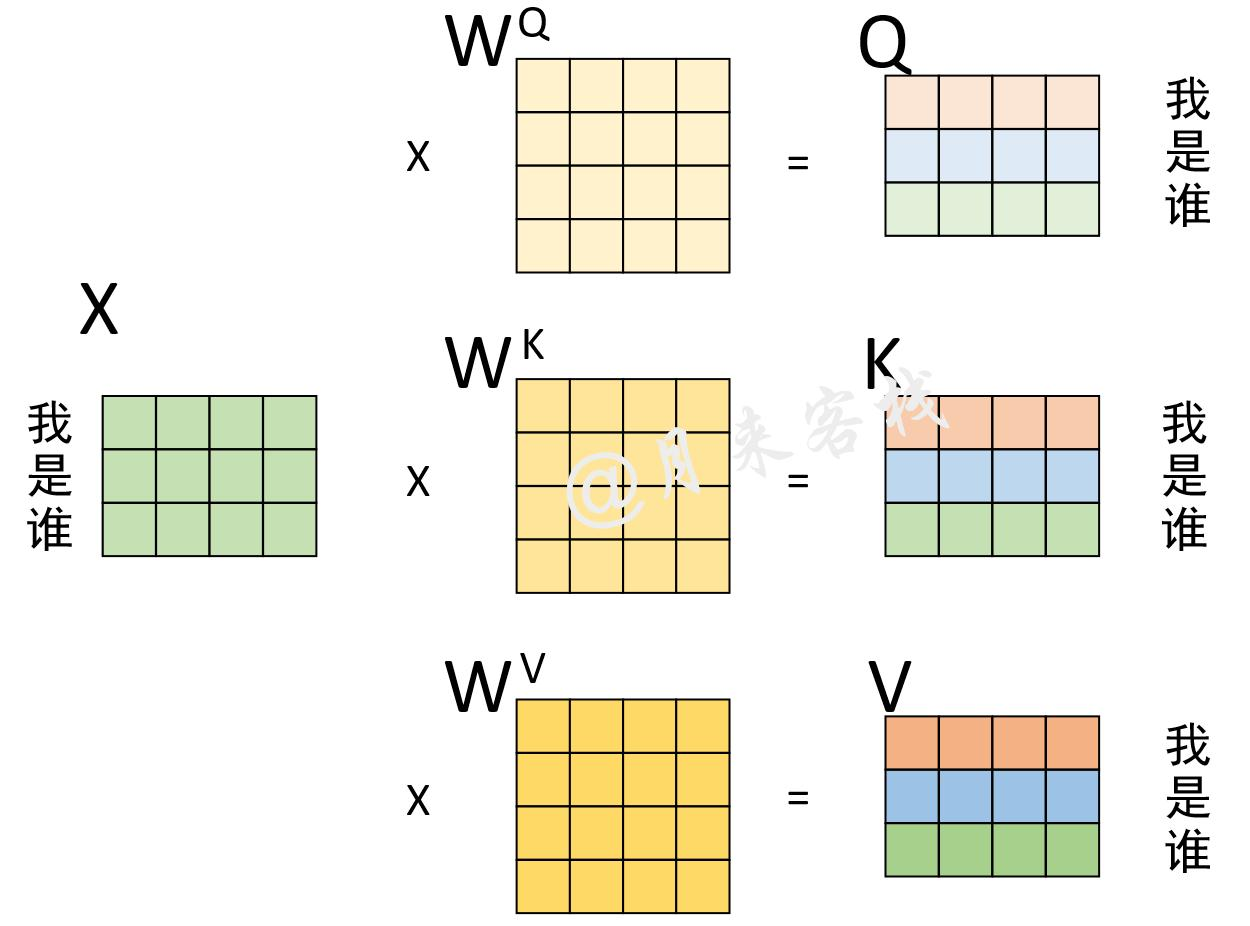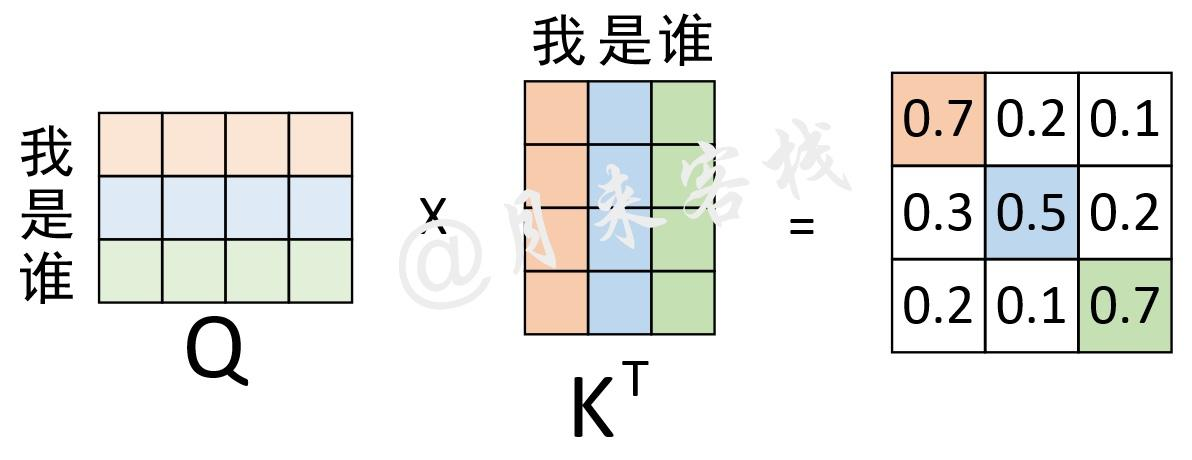It expands the model’s ability to focus on different positions. Yes, in the example above, z1 contains a little bit of every other encoding, but it could be dominated by the the actual word itself.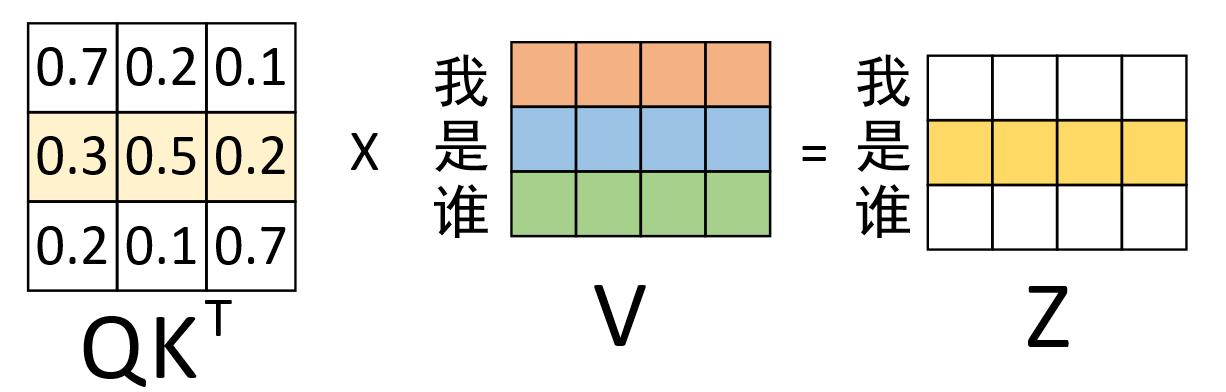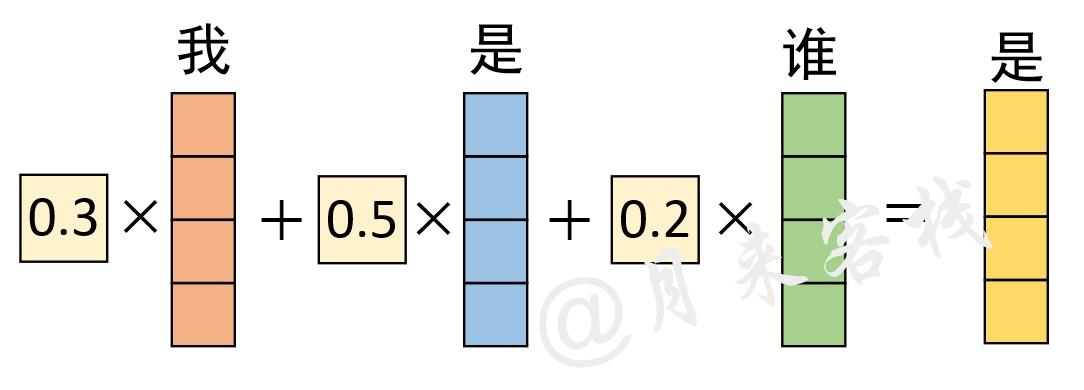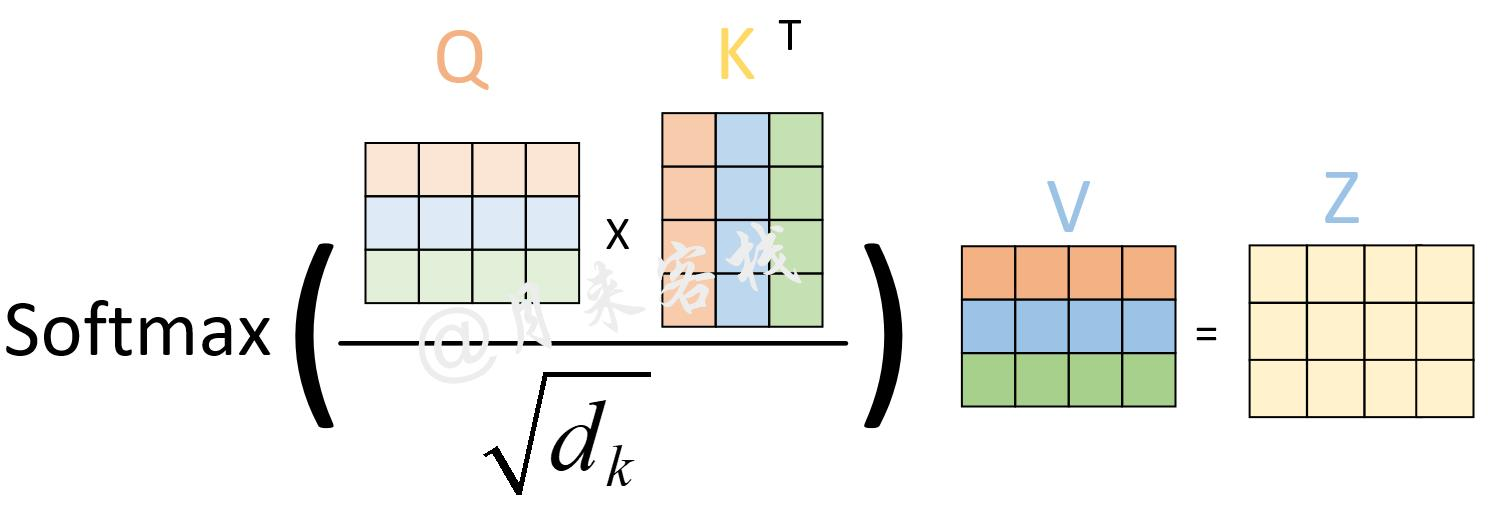Multi-head attention allows the model to jointly attend to information from different representation subspaces at different positions.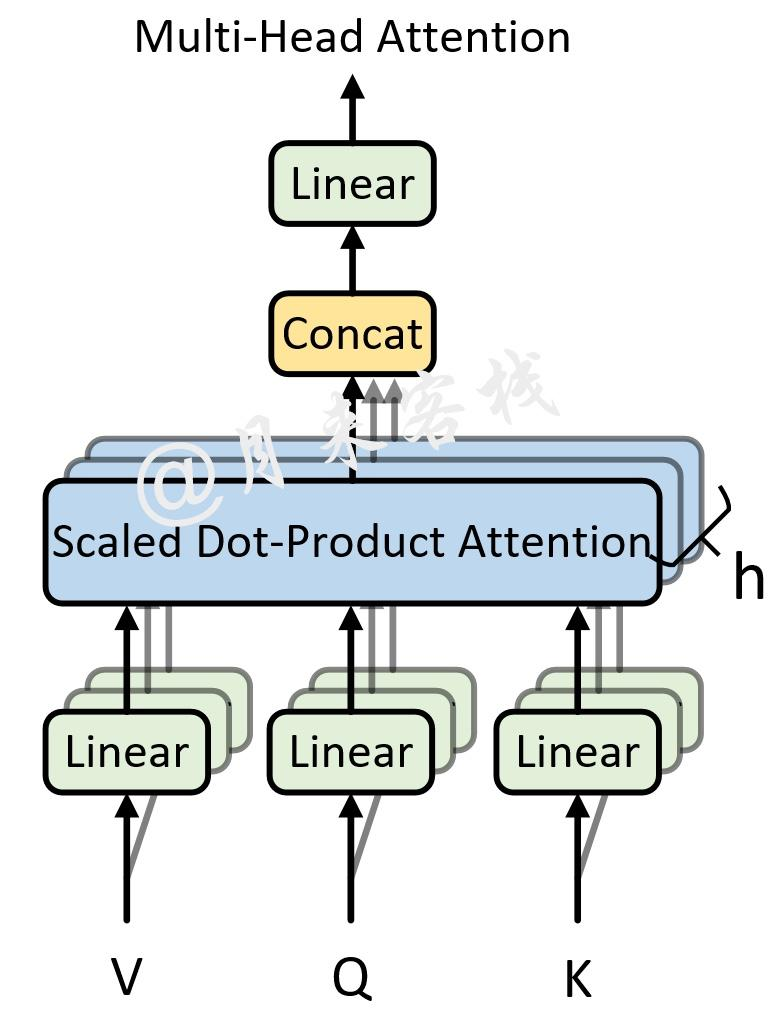\begin{array}{c}
where \operatorname{head}_{i} = \operatorname{Attention}\left(Q W_{i}^{Q}, K W_{i}^{K}, V W_{i}^{V}\right)
\end{array}

W_{i}^{Q} \in \mathbb{R}^{d_{\text {model }} \times d_{k}}, W_{i}^{K} \in \mathbb{R}^{d_{\text {model }} \times d_{k}}, W_{i}^{V} \in \mathbb{R}^{d_{\text {model }} \times d_{v}}, W^{O} \in \mathbb{R}^{h d_{v} \times d_{\text {model }}}